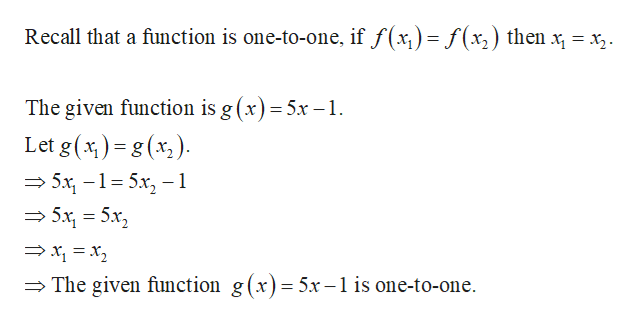# Given g(x) = 5x− 1,a. Is g a one-to-one function?b.Write an equation for g−1(x).

Question
4 views

Given g(x) = 5x− 1,

a. Is g a one-to-one function?

b.Write an equation for g−1(x).

check_circle

Step 1

(a) Check whether the given function i...help_outlineImage TranscriptioncloseRecall that a function is one-to-one, if f(x,)=f(x2) then x, = x . The given function is g (x) = 5x -1. %3D Let g(x ) = g (x,). %3D — 5х, -1-5х, —-1 %3D = 5x, = 5x, → x, = x, = The given function g(x)= 5x – 1 is one-to-one. fullscreen

### Want to see the full answer?

See Solution

#### Want to see this answer and more?

Solutions are written by subject experts who are available 24/7. Questions are typically answered within 1 hour.*

See Solution
*Response times may vary by subject and question.
Tagged in

### Algebra